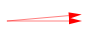### Home > MC1 > Chapter 8 > Lesson 8.1.1 > Problem8-19

8-19.

Without measuring, draw an angle that has approximately each of the measures shown below. Explain how you decided how big the angle should be.

1.  $45^\circ$

$45$ is half of $90$.

1.  $5^\circ$1.  $95^\circ$

$95$ is slightly larger than $90$.

1.  $175^\circ$

$175$ is slightly less than $180$.